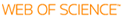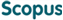BROWSE

Related ResearcherLee, Chang Hyeong
Stochastic Analysis & Simulation(SAS) Lab
Research Interests
• Stochastic analysis/computation, epidemic modeling, biological system simulation

A numerical characteristic method for probability generating functions on stochastic first-order reaction networks

Cited 0 times inCited 0 times inTitle
A numerical characteristic method for probability generating functions on stochastic first-order reaction networks
Author
Issue Date
2013-01
Publisher
SPRINGER
Citation
JOURNAL OF MATHEMATICAL CHEMISTRY, v.51, no.1, pp.316 - 337
Abstract
We propose an efficient and accurate numerical scheme for solving probability generating functions arising in stochastic models of general first-order reaction networks by using the characteristic curves. A partial differential equation derived by a probability generating function is the transport equation with variable coefficients. We apply the idea of characteristics for the estimation of statistical measures, consisting of the mean, variance, and marginal probability. Estimation accuracy is obtained by the Newton formulas for the finite difference and time accuracy is obtained by applying the fourth order Runge-Kutta scheme for the characteristic curve and the Simpson method for the integration on the curve. We apply our proposed method to motivating biological examples and show the accuracy by comparing simulation results from the characteristic method with those from the stochastic simulation algorithm.
URI
https://scholarworks.unist.ac.kr/handle/201301/2859
URL
DOI
10.1007/s10910-012-0085-8
ISSN
0259-9791
Appears in Collections:
MTH_Journal Papers
Files in This Item:
There are no files associated with this item.